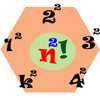Search by Topic

Resources tagged with Factorials similar to Tens:

Filter by: Content type:
Age range:
Challenge level:

There are 10 results

Broad Topics > Numbers and the Number System > FactorialsGrowing

Age 16 to 18 Challenge Level:

Which is larger: (a) 1.000001^{1000000} or 2? (b) 100^{300} or 300! (i.e.factorial 300)Squaresearch

Age 14 to 16 Challenge Level:

Consider numbers of the form un = 1! + 2! + 3! +...+n!. How many such numbers are perfect squares?Fac-finding

Age 14 to 16 Challenge Level:

Lyndon chose this as one of his favourite problems. It is accessible but needs some careful analysis of what is included and what is not. A systematic approach is really helpful.Factorial

Age 14 to 16 Challenge Level:

How many zeros are there at the end of the number which is the product of first hundred positive integers?The Harmonic Triangle and Pascal's Triangle

Age 16 to 18

The harmonic triangle is built from fractions with unit numerators using a rule very similar to Pascal's triangle.Seriesly

Age 16 to 18 Challenge Level:

Prove that k.k! = (k+1)! - k! and sum the series 1.1! + 2.2! + 3.3! +...+n.n!Factorial Fun

Age 16 to 18 Challenge Level:

How many divisors does factorial n (n!) have?Seriesly

Age 16 to 18 Short Challenge Level:

Prove that k.k! = (k+1)! - k! and sum the series 1.1! + 2.2! + 3.3! +...+n.n!N000ughty Thoughts

Age 14 to 16 Challenge Level:

How many noughts are at the end of these giant numbers?